# Quantitative Analysis of Non-Linear High Entropy Economic Systems VII

From: John Conover <john@email.johncon.com>
Subject: Quantitative Analysis of Non-Linear High Entropy Economic Systems VII
Date: 28 Aug 2006 09:39:47 -0000

### Introduction

As mentioned in Section I, Section II, Section III, Section IV, Section V and Section VI, much of applied economics has to address non-linear high entropy systems-those systems characterized by random fluctuations over time-such as net wealth, equity prices, gross domestic product, industrial markets, etc.

### Review

A quick review of this series.

Many economic systems are characterized by non-linear high entropy time series. These time series are a geometric progression, as analyzed in Section I, and the distribution of the marginal increments of the time series exhibit log-normal distributions, as suggested in Section II. The characteristics of the marginal increments can be analyzed as suggested in Section III, and, Section IV, to formulate investment strategies and optimizations as illustrated in Section V. The finer details of the types of leptokurtosis found in the marginal increments of financial time series is analyzed in Section VI.

### A concluding example, the DJIA

Revisiting the DJIA, (since it has a long historical database,) a meticulous analytical approach will be used to analyze the characteristics of the closing values of the DJIA. The analytical procedure will use a conscientious process commonly used in engineering practice:

1. Assume a systemic model, (in this case, that the time series is a geometrical progression.)
2. Extract/analyze the values of the variables used in the model. This will be done with a `script` of analytical programs, "chained" together, (usually with Unix pipes for maintainability and extensibility.)
3. The variables will be used to simulate the characteristics of the systemic model.
4. The empirical data and simulated data will be compared, using analytical programs, and pictographic presentations, (i.e., graphs,) to provide an intuitive interpretation of the data, and its comparison to the theoretical model-in every step in the analysis.

Note: the C source code to all programs used in the `script` are available from the NtropiX Utilities page, or, the NdustriX Utilities page, and is distributed under License.

The historical time series of the DJIA index was obtained from Yahoo!'s database of equity Historical Prices, (ticker symbols ^DJI,) in csv format. The csv format was converted to a Unix database, `djia`, using the `csv2tsinvest` program. (The DJIA time series started on January 2, 1900, and contained 29010 daily closes, through May 26, 2006.)

Plotting the closing values of the DJIA: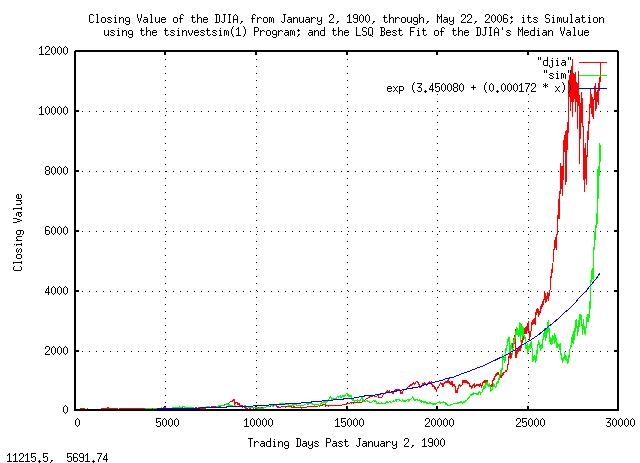Figure I

Figure I is a plot of the daily closes of the DJIA, from January 2, 1900, through, May 26, 2006. The simulated value is constructed from the variables extracted from the empirical data in the `script`, below, as is the median value, and presented here for comparison.

The `script` used for the programs will be walked through statement by statement, to illustrate and validate the analytic procedure.

Starting with the first two statements, and following the outline from Section I:

``````

tsfraction djia | tsavg -p
0.000236
tsfraction djia | tsrms -p
0.010950

``````

From Equation (1.24), `P = 0.51077625570776255708`, meaning that there are, on average, about `51` up movements, and `49` down movements, out of one hundred. `P` is the probability of an up movement in the DJIA.

Log-normal distributions of the marginal increments of a time series-those distributions commonly found in geometric progressions-are difficult to comprehend intuitively, and it is expedient to convert the time series to its Brownian Motion, (random walk,) equivalent as outlined in Section II.

The root-mean-square, `rms`, of the Brownian Motion equivalent, (the next two statements in the `script`):

``````

tsfraction djia | tsmath -s 0.000236 | tsrms -p
0.010947
tsmath -l djia | tsderivative | tsmath -s 0.000176 | tsrms -p
0.010998

``````

which are alternative methods-the first extracts the `rms` directly from the geometric progression, and the second from its Brownian Motion equivalent. The two answers should be nearly identical. The offset, ```avg = 0.000236```, is subtracted from the first, and `ln (g) = ln (1.000176) = 0.000176` from the second. The logarithm of the `rms` will be useful later, ```ln (0.010947) = -4.51468983285971677053```.

The number of elements in the time series, and its beginning value will be of interest, later:

``````

wc djia
29010  29010 202761 djia
68.13

``````

The marginal gain, `g` of the Brownian Motion equivalent is determined by the next two statements in the `script`:

``````

tsgain -p djia
1.000176
tsmath -l djia | tsderivative | tsavg -p
0.000176

tslsq -e -p djia
e^(3.450080 + 0.000172t) = 1.000172^(20062.070643 + t) = 2^(4.977413 + 0.000248t)

``````

The two answers should be nearly equivalent. The third line in this section of the script provides yet another method-it uses the exponential Least-Squares, (LSQ,) best fit to the original time series; it, too, should provide a nearly identical answer to the to the other two methods, (`0.000176` vs. `0.000172`.) The LSQ best fit to the data starts with a first element value of ```exp (3.450080) = 31.50291244093657542517```.

Using the variables produced by the LSQ best-fit, and plotting the Brownian Motion equivalent of the DJIA: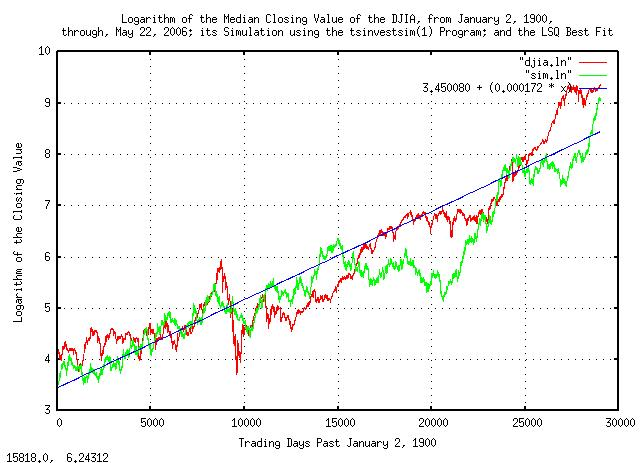Figure II

Figure II is a plot of the Brownian Motion, (random walk,) equivalent of the DJIA, from January 2, 1900, through, May 26, 2006. The simulated values are constructed from the variables extracted from the empirical data in the `script`, below.

Having converted the DJIA's time series to its Brownian Motion equivalent, the marginal increments can be analyzed. One of issues to be addressed is leptokurtosis-specifically, the deviation from the theoretical assumption that the increments are statistically independent-this will indicate what math should be used, (if the increments are independent, then root-mean-square should be used, if not, another root-mean should be used, as per Section VI.) An iterated script will be used to find the root:

``````

R="0.5"
#
> "log"
#
LAST="NOTHING"
#
LOOP="1"
#
while [ "\${LOOP}" -eq "1" ]
do
tsmath -l djia | tsderivative | tsmath -s 0.000176 | tsintegrate | \
tsrunmagnitude -r "\${R}" > "djia.magnitude"
cut -f1 "djia.magnitude" | tsmath -l > "temp.5"
cut -f2 "djia.magnitude" | tsmath -l > "temp.6"
LAST=`paste temp.5 temp.6 | egrep '^[0-5]\.' | tslsq -p`
echo "\${LAST}"
R=`echo "\${LAST}" | sed -e 's/^.* //' -e 's/t.*\$//'`
#
if grep -e "\${LAST}" "log"
then
LOOP="0"
fi
#
mv "temp.5" "temp.5.last"
mv "temp.6" "temp.6.last"
mv "djia.magnitude" "djia.magnitude.last"
echo "\${LAST}" >> "log"
done

``````

The `script` fragment is an iterated search-for-solution algorithm that initially assumes a root of `0.5`, uses `tsrunmagnitude` to analyze the time series and produce a more accurate approximation to the root, and so on, until no further improvements were possible. (The other statements in the loop are standard Unix text database manipulations, using `cut(1)` and `paste(1)` to extract, and reassemble fields in the database, `egrep(1)` to extact only days `1 - e^5.999... = 403` days, and so forth.)

The output of the `script` fragment is:

``````

-4.592316 + 0.537435t
-4.648576 + 0.541035t
-4.653584 + 0.541347t
-4.654019 + 0.541375t
-4.654057 + 0.541377t
-4.654058 + 0.541377t
-4.654058 + 0.541377t

``````

meaning that, at least in the very short term, (i.e., daily returns,) there is about a `54%` chance that what happened on any one day will occur on the next day, also.

The simulation can now be constructed using the `tsinvestsim` program with the file, `djia.sim`:

``````

djia, p = 0.51077625570776255708, f = 0.010950, i = 31.50291244093657542517, h = 0.541377, l = 1

``````

and running the `tsinvestsim`:

``````

tsinvestsim djia.sim 29010 | cut -f3 > sim

``````

And, analyzing the simulation file, `sim`, in an identical manner to the DJIA analysis:

``````

tsfraction sim | tsavg -p
0.000253
tsfraction sim | tsrms -p
0.010994
tsmath -l sim > sim.ln

tslsq -e -p sim
e^(3.548001 + 0.000146t) = 1.000146^(24382.768809 + t) = 2^(5.118683 + 0.000210t)

``````

Which compares favorably to the original analysis of the DJIA. The files produced in the simulation were presented in Figure I and Figure II, above, for comparison with the original DJIA time series.

The ground work is now prepared to look into issues of leptokurtosis of the DJIA. As presented in Section VI, the model used will be Laplacian distribution:

``````

tsfraction djia | tsmath -s 0.000236 | tsnormal -t > djia.distribution
tsfraction djia | tsmath -s 0.000236 | tsnormal -t -f > djia.frequency
tsfraction sim | tsmath -s 0.000236 | tsnormal -t > sim.distribution
tsfraction sim | tsmath -s 0.000236 | tsnormal -t -f > sim.frequency

egrep '^-' djia.frequency | wc
50     100     950
egrep '^-' djia.frequency | tail -49 | tslsq -e -p | sed 's/ = .*\$//'
e^(0.710298 + 147.146009t)

``````

Here, the offset of distribution is subtracted, as above, from the marginal increments of the DJIA's value, and its simulation, and a histogram of the marginal increments made with the `tsnormal` program. An LSQ approximation to the distribution is necessary, and since the Laplace distribution is a double exponential, the negative side of the distribution is omitted using `egrep(1)`, and the `tslsq` program used to provide the LSQ best-fit approximation to the distribution. And plotting: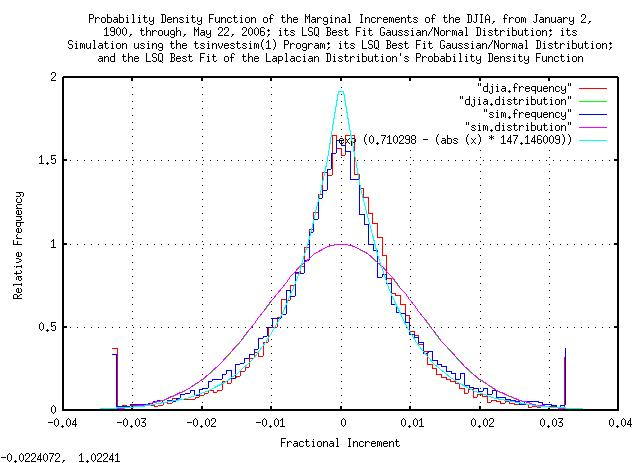Figure III

Figure III is a plot of the distribution of the marginal increments of the Brownian Motion, (random walk,) equivalent of the DJIA, from January 2, 1900, through, May 26, 2006, and its simulation. The Gaussian/Normal LSQ best-fit approximation is presented as a comparison, also-the variance of all distributions shown is nearly identical, as would be expected.

Integrating the count of marginal increments in each `0.1%` "bucket" to obtain the cumulative probabilities:

``````

tsfraction djia | tsmath -s 0.000236 | sed 's/[0-9][0-9][0-9]\$//' | sort -n | \
tscount -r | tsmath -t -d 29009 | tsintegrate -t > djia.cumulative
tsfraction sim | tsmath -s 0.000236 | sed 's/[0-9][0-9][0-9]\$//' | sort -n | \
tscount -r | tsmath -t -d 29009 | tsintegrate -t > sim.cumulative

``````

And plotting:Figure IV

Figure IV is a plot of the cumulative distribution of the marginal increments of the Brownian Motion, (random walk,) equivalent of the DJIA, from January 2, 1900, through, May 26, 2006, and its simulation. It was analyzed by a different method-its derivative should be much the same as Figure III, above, and is included as a method of cross-checking the data and analysis.

The run lengths of the expansions and contractions of the DJIA:

``````

tsmath -l djia | tsderivative | tsmath -s 0.000176 | tsintegrate | tsrunlength | cut -f1,7 > djia.length
tsmath -l sim | tsderivative | tsmath -s 0.000176 | tsintegrate | tsrunlength | cut -f1,7 > sim.length

``````

And, plotting: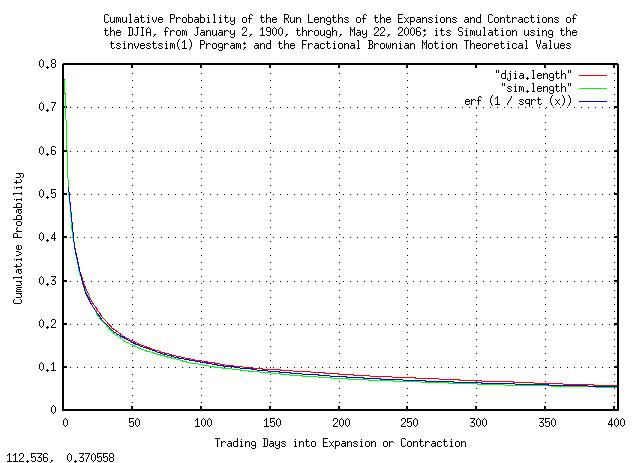Figure V

Figure V is a plot of the cumulative probability of the run lengths of the expansions and contractions of the Brownian Motion, (random walk,) equivalent of the DJIA, from January 2, 1900, through, May 26, 2006, and its simulation. ```erf (1 / sqrt (x))``` is the theoretical value. As an example interpretation, there is a little over `10%` chance of a the value of the DJIA being above its median value for at least `100` trading days.

And, the magnitude of the expansions and contractions of the DJIA:

``````

tsmath -l djia | tsderivative | tsmath -s 0.000176 | tsintegrate | tsrunmagnitude > djia.magnitude
tsmath -l sim | tsderivative | tsmath -s 0.000176 | tsintegrate | tsrunmagnitude > sim.magnitude

``````

And, plotting: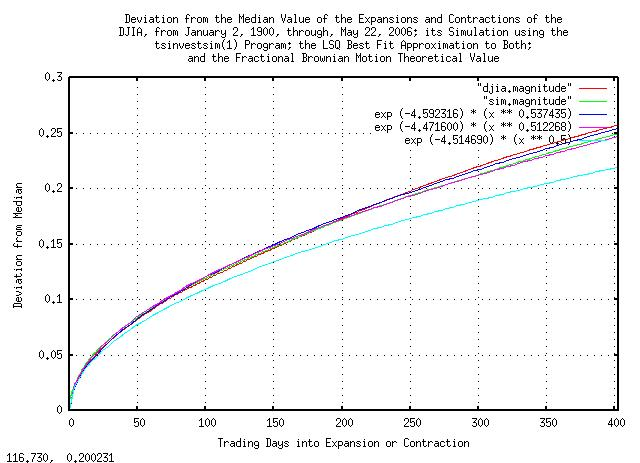Figure VI

Figure VI is a plot of the deviation from the median value of the expansions and contractions of the Brownian Motion, (random walk,) equivalent of the DJIA, from January 2, 1900, through, May 26, 2006, and its simulation. `0.010947 * sqrt (x)` is the theoretical value. As an example interpretation, there is a standard deviation chance that the value of the DJIA will be within a little more than `+/- 10%` of its median value at `100` trading days.

The discrepancies of the curves from the theoretical values are do to market inefficiencies. The empirical curves are steeper for small time intervals, (near 1 day,) because the market does not respond instantaneously to new information-there is a slight persistence from one day to the next. Additionally, the empirical curves are steeper than the theoretical at `253` trading days, (about a calendar year,) for structural reasons-specifically, taxation schedules that favor funds selling off losing equities before the end of the calendar year. It should be noted that deviation from the theoretical values is not constant, and varies throughout the calendar year. The LSQ best fit approximations are an average over the `403` days-about `19` months.

Market inefficiencies are exploitable, (if the DJIA were a perfect Brownian Motion random walk, the market would be fair, and no one could have an advantage over anyone else in the long run.) Delving into the market inefficiencies by making a log-log plot of Figure VI.

``````

cut -f1 djia.magnitude | tsmath -l > temp.1
cut -f2 djia.magnitude | tsmath -l > temp.2
paste temp.1 temp.2 > djia.magnitude.ln

cut -f1 sim.magnitude | tsmath -l > temp.3
cut -f2 sim.magnitude | tsmath -l > temp.4
paste temp.3 temp.4 > sim.magnitude.ln

egrep '^[0-5]\.' djia.magnitude.ln | tslsq -p
-4.592316 + 0.537435t
egrep '^[0-5]\.' sim.magnitude.ln | tslsq -p
-4.471600 + 0.512268t

``````

And, plotting: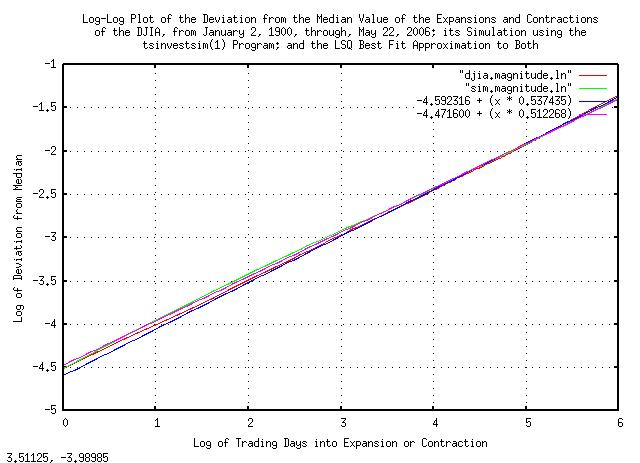Figure VII

Figure VII is a log-log plot of the deviation from the median value of the expansions and contractions of the Brownian Motion, (random walk,) equivalent of the DJIA, from January 2, 1900, through, May 26, 2006, and its simulation shown in Figure VI.

And, plotting Figure VII for short time intervals to emphasize the market inefficiency: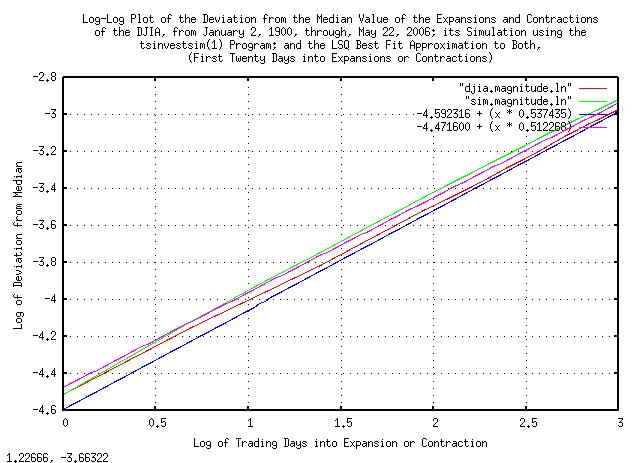Figure VIII

Figure VIII is a log-log plot of the deviation from the median value of the expansions and contractions of the Brownian Motion, (random walk,) equivalent of the DJIA, from January 2, 1900, through, May 26, 2006, and its simulation, plotted for a few trading days.

And, plotting Figure VII around a calendar year to emphasize the market inefficiency: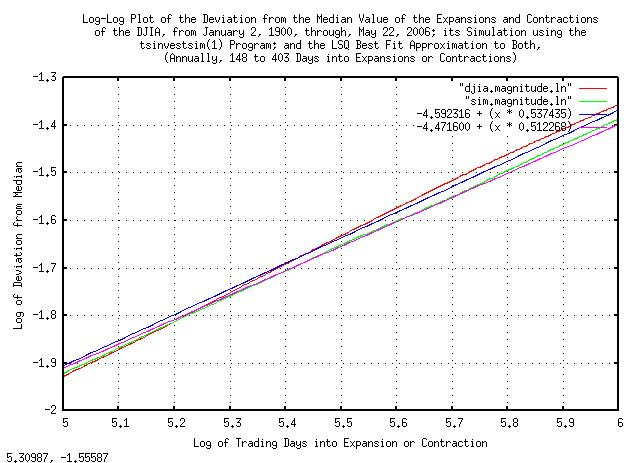Figure IX

Figure IX is a log-log plot of the deviation from the median value of the expansions and contractions of the Brownian Motion, (random walk,) equivalent of the DJIA, from January 2, 1900, through, May 26, 2006, and its simulation, plotted at a calendar year.

Figure VIII and Figure IX indicate exploitable market inefficiencies-where the marginal increments are not statistically independent, (iid,) meaning some sense of predictability.

To remove the statistical dependence, the marginal increments of the Brownian Motion, (random walk,) equivalent of the DJIA can be moved randomly, (i.e., scrambled,) in the time series, and the random walk equivalent of the time series re-assembled, then the deviation from the median value of the expansions and contractions analyzed:

``````
#
tsmath -l djia | tsderivative | tssequence | sort -n | cut -f3 | \
tsmath -s 0.000176 | tsintegrate > "scrambled"
#
R="0.5"
#
> "log"
#
LAST="NOTHING"
#
LOOP="1"
#
while [ "\${LOOP}" -eq "1" ]
do
tsrunmagnitude -r "\${R}" "scrambled" > "scrambled.magnitude"
cut -f1 "scrambled.magnitude" | tsmath -l > "temp.7"
cut -f2 "scrambled.magnitude" | tsmath -l > "temp.8"
LAST=`paste temp.7 temp.8 | egrep '^[0-5]\.' | tslsq -p`
echo "\${LAST}"
R=`echo "\${LAST}" | sed -e 's/^.* //' -e 's/t.*\$//'`
#
if grep -e "\${LAST}" "log"
then
LOOP="0"
fi
#
mv "temp.7" "temp.7.last"
mv "temp.8" "temp.8.last"
mv "scrambled.magnitude" "scrambled.magnitude.last"
echo "\${LAST}" >> "log"
done

``````

The output of the `script` fragment is:

``````

-4.498716 + 0.496851t
-4.495234 + 0.496649t
-4.495005 + 0.496635t
-4.494994 + 0.496635t
-4.494994 + 0.496635t
-4.494994 + 0.496635t

``````

And, plotting: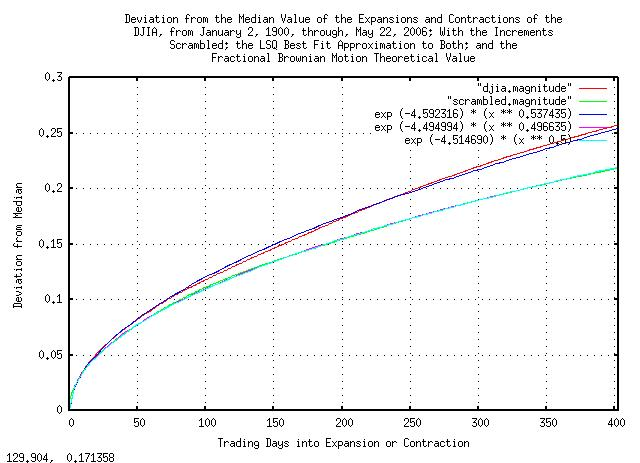Figure X

Figure X is a plot of the deviation from the median value of the expansions and contractions of the scrambled Brownian Motion, (random walk,) equivalent of the DJIA, from January 2, 1900, through, May 26, 2006, and its simulation. ```0.010947 * sqrt (x)``` is the theoretical value. Note that comparing with Figure VI, the deviation is, within numerical precision, very close the the theoretical value.

The distribution of the marginal increments of the scrambled Brownian Motion, (random walk,) equivalent of the DJIA are the same as shown in Figure III, above, since they are the same increments.

How good is the Laplacian distributed marginal increment approximation?

To get an idea, compare the PDF tail with the empirical tail using the formula for the PDF:

``````

exp (0.710298 - (abs (x) * 147.146009))

``````

The deviation would be:

``````

sqrt (2) / 147.146009 = 0.009610954

``````

There were `29015` trading days represented in the time series for the DJIA, and ```1 / 29015 = 0.000034464```, so, the value `0.07466`, (`7.7682` deviations, the largest expected marginal increment in the PDF,) should be represented in the time series about once, (e.g., ```exp (0.710298 - (abs (0.07466) * 147.146009)) = 0.000034464```). Any more would be excess "fat tails," which the model did not handle appropriately. Sorting by the value of marginal increments:

``````

tsfraction -t "djia" | cut -f1 > "1.temp"
tsfraction -t "djia" | cut -f2 > "2.temp"
paste "2.temp" "1.temp" | sort -n > "djia.increments"

``````

And editing for those marginal increment values greater than `0.07466`:

Value Date

-0.235228

19141213

-0.226105

19871019

-0.128207

19291028

-0.117288

19291029

-0.113283

19211219

-0.105631

19180920

-0.099154

19291106

-0.087304

19330526

-0.084035

19320812

-0.082892

19070314

-0.080394

19871026

-0.078433

19330721

-0.077550

19371018

0.079876

19320610

0.087032

19311008

0.090343

19330419

0.090758

19320506

0.091858

19320213

0.093509

19311218

0.093563

19291114

0.094708

19320211

0.095184

19320803

0.101488

19871021

0.106771

19330531

0.113646

19320921

0.118652

19180919

0.118839

19211217

0.123441

19291030

0.153418

19330315

TABLE I.

Table I is a list of the `29` marginal increments of the DJIA, (January 2, 1900, to, May 22, 2006, inclusive,) that were greater than `0.07466`, (`7.7682` deviations.) The `29` represent ```29 / 29015 = 0.00099948``` or about `0.1%`, which would be expected about once every `1000` trading days, or about once every `4` years of `253` trading days per year.

Finding the marginal increments that were greater than `0.07466` by year:

``````

cut -f2 "djia.increments" | sed 's/[0-9][0-9][0-9][0-9]\$//' | \
sort -n | tscount | sort -n

``````
Number in Year Year

1

1907

1

1914

1

1937

2

1918

2

1921

2

1931

3

1987

5

1929

5

1933

7

1932

TABLE II.

Table II is a list of the `29` marginal increments of the DJIA, (January 2, 1900, to, May 22, 2006, inclusive,) that were greater than `0.07466`, (`7.7682` deviations,) by year in which the excessive marginal increment occurred. Notice the extreme clustering in the Great Depression; if it was a random process, we would expect to see the excessive increments about once every four years, yet 1932 had seven, and there were seventeen between 1929 and 1933, an order of magnitude and a half too many.

Hand editing for the month in which excessive marginal increments occurred:

Number in Month Month

1

04

1

06

1

07

2

02

2

03

2

08

2

11

3

05

3

09

4

12

8

10

TABLE III.

Table III is a list of the `29` marginal increments of the DJIA, (January 2, 1900, to, May 22, 2006, inclusive,) that were greater than `0.07466`, (`7.7682` deviations,) by month in which the excessive marginal increment occurred. Notice the clustering in October; if it was a random process, we would expect to see the excessive increments about ```29 / 12 = 2.417``` times a month, yet October had eight-about a factor of 3 too many, (the beginning of calendar Q4 is when fund managers-managing about 60% of equities in the US equity markets-sell off their losers for the year for tax purposes; so this may be a structural issue.)

It is doubtful that an analytic function PDF with a finite variance, (even if stable,) will model the clustered excessive increments adequately-and addressing the issues will have to be left to experienced judgment. It is worth noting, however, that the market does correct itself of excessive increments; the sum of the negative excessive increments, (`13` of them,) in the DJIA's daily close for `106` years is `-1.515504`; the sum of the positive excessive increments, (`16` of them,) is `1.653086`, almost totally cancelling, in the extreme long run. (Or, as a geometric progression, the negative excessive marginal increments was `0.195027`, and the positive `4.811824`, for a factor of `0.195027 * 4.811824 = 0.9384`, which is remarkable considering the DJIA increased by a factor of `11125.32 / 68.13 = 163.30` in the `106` years.) It may well be that a survival strategy for a once-in-four years excessive marginal increment cluster is appropriate, (but that is not easy; for example, during the Great Depression, the DJIA was a maximum of 381.17 on September 3, 1929, and deteriorated to a minimum for the century of 41.22 on July 8, 1932-about 3 years later-and did not recover to its original maximum until November 23, 1954 when it hit 382.74-about a quarter of a century later; and that is not adjusted for inflation.)

The annual market inefficiencies would be difficult to exploit, (they only happen once a year,) except as a defensive strategy. However, the short term inefficiencies do offer an opportunity. Rerunning the `script` with an LSQ of only a few days:

``````

R="0.5"
#
> "log"
#
LAST="NOTHING"
#
LOOP="1"
#
while [ "\${LOOP}" -eq "1" ]
do
tsmath -l djia | tsderivative | tsmath -s 0.000176 | tsintegrate | \
tsrunmagnitude -r "\${R}" > "djia.magnitude"
cut -f1 "djia.magnitude" | tsmath -l > "temp.9"
cut -f2 "djia.magnitude" | tsmath -l > "temp.10"
LAST=`paste temp.9 temp.10 | egrep '^\.' | tslsq -p`
echo "\${LAST}"
R=`echo "\${LAST}" | sed -e 's/^.* //' -e 's/t.*\$//'`
#
if grep -e "\${LAST}" "log"
then
LOOP="0"
fi
#
mv "temp.9" "temp.9.last"
mv "temp.10" "temp.10.last"
mv "djia.magnitude" "djia.magnitude.last"
echo "\${LAST}" >> "log"
done

``````

The output of the `script` fragment is:

``````

-4.510133 + 0.518242t
-4.539663 + 0.521663t
-4.545016 + 0.522309t
-4.546053 + 0.522481t
-4.546241 + 0.522468t
-4.546241 + 0.522468t
-4.546241 + 0.522468t

``````

Meaning that there is a little over a 2% chance that what happened in the DJIA on any given day will happen on the next day, also.

This analysis was originally used to design the algorithm used in the `-d5` option to the `tsinvest` program. Checking:

``````

sed 's/^/DJIA      /' djia | tsnumber | tsinvest -r | tail -1
# DJIA, p = 0.510810, f = 0.010949, h = 0.544745, i = 68.130000

tsinvestsim djia.sim 29010 | tsinvest -r | tail -1
# DJIA, p = 0.511489, f = 0.010994, h = 0.548321, i = 31.787033

``````

Numbers which agree very favorably with this analysis. And, running the program on the DJIA time series, from January 2, 1900, through, May 26, 2006:

``````

sed 's/^/DJIA    /' djia | tsnumber | tsinvest -its -d5 | egrep DJIA | cut -f3 | tsgain -p
1.000535

``````

The theoretical gain, `g`, per trading day would be, (from: Equation (1.20)):

``````

rms = e^(-4.546241) = 0.0106070013
P = 0.522468
g = ((1 + 0.0106070013)^0.522468) * ((1 - 0.0106070013)^(1 - 0.522468))
g = 1.0004204851

``````

The reason the measured daily gain, `g`, is larger than the theoretical value is the sophistication of the algorithm used in the `tsinvest` program-it maintains two different tables, (one probability density function for positive movements, another for negative,) and calculates the probabilities of future movements using the empirically derived probability density functions, (as opposed to the LSQ approximation of daily returns for a year used in this analysis.) But the theoretical and empirical values are reasonably close.

Compare these values with the gain of the DJIA, from January 2, 1900, through, May 26, 2006:

``````

tsgain -p djia
1.000176

``````

Which would be the long term investment potential of the DJIA, (from Equation (1.24)):

``````

avg = 0.000236
rms = 0.010950

P = ((0.000236 / 0.010950) + 1) / 2 = 0.51077626

g = ((1 + 0.010950)^0.51077626) * ((1 - 0.010950)^(1 - 0.51077626))
g = 1.0001760701

``````

The difference in annual gain is significant. Exploiting short term market inefficiencies resulted in an annual gain, (of 253 trading days,) of ```1.000535^253 = 1.1449017271```, or a little less than 15% per year. Compared with ```1.000176^253 = 1.0455301549```, or a little less than 5% per year as a long term investment.

There are other engineered solutions for increasing the value of investments in the DJIA equities, too-as explained in Quantitative Analysis of Non-Linear High Entropy Economic Systems V-specifically, see a simulation of the strategy, which yielded a little over a 17% annual growth in value over the last quarter of the Twentieth Century.

It is interesting to note that, in the long run, a well executed long term portfolio strategy-specfically, rebalancing expeditiously-is more important than timing the market, (which is what this analysis was about,) which, in turn, is more important than picking winners.

A well designed strategy does all three, but in that order of priority.

 As a side bar, this is the intended usage of the `tsinvest` program-it is, essentially, an automated broker. It assembles and maintains a portfolio from a universe of equities, (thousands are common,) according to a strategy, as defined on the command line. The universe of equities is typically a stock ticker, (but can be an historical database of the ticker for research.) The program is typically used on equities, but is not restricted to equities alone-different investments can be mixed and matched, (for example, optimizing the balance of money between a saving account and a portfolio of equities, and/or properties, dynamically.) A word of caution, however. The program is a tool, and a tool is no better than the mechanic using it. It is not a substitute for due diligence and meticulous research. It would probably be better to view the program as a search mechanism for investments-like a Google of the ticker, where one searches for equities/investments that fit a search criteria, (i.e., an investment strategy.) It is a tool for extending the depth and breadth, (and speed,) of investing.

A note about the DJIA time series:

• From 1895, the inception of the DJIA, (although this analysis started with January 2, 1900,) until 1953, trading used 6 day, (actually, five and a half-but even that varied,) weeks-after 1953, 5 day weeks were used; about half of the century's data had 6/5's as many trading days per year in the time series. The number of holidays when the exchanges closed varied over the century, too.

• Prior to 2001, equity values were listed in fractions of 1/8'th dollar, (i.e., from the pieces of eight tradition of the NYSE.) After 2001, values were expressed in decimal values, i.e., 1/100'th of a dollar, or a penny.

• The DJIA is made up of 30 equities, and the equities are changed to represent the market environment; for example, only GE has a been a constituent part of the DJIA since its inception.

• The market inefficiencies have evolved over the century due to the advent of programmed/algorithmic trading. At the beginning of the Twentieth Century, brokers could make handsome profits exploiting the spread, (i.e., arbitrage-since the markets moved at a slower pace,) but at the end of the century, the spread was so small that many brokerage firms had to seek other endeavors-like selling analysis of companies and their equities.

All of these represent anomalies effecting the accuracy of the analysis.

The time series of the DJIA contained 29010 daily closes, (29009 increments.) The margin of error, (using statistical estimation,) would be ```0.010950 / sqrt (29009) = 0.0000642906```, meaning that there is a 95% probability, (i.e., two double sided standard deviations,) that the deviation of the increments is more than ```0.010950 - 0.0000642906 = 0.0108857094```, and, less than ```0.010950 + 0.0000642906 = 0.011014291```, which is about `+/- 0.6%`. There is, also, a 95% probability that the average of the increments is more than `0.000236 - 0.0000642906 = 0.0001717094`, and, less than ```0.000236 + 0.0000642906 = 0.0003002906```, which is a little more than `+/- 27%`, which could be a source of significant error in the analysis-the average of the increments can only be known to within a factor of about 2, with a 95% confidence level. (Note that this uncertainty can be addressed by modifying `P` in Equation (1.24) appropriately to accommodate data set size issues. This is how the `tsinvest` program avoids "chasing bubbles"; its just another uncertainty that the program has to address).

The distribution of the increments of the Brownian Motion, (random walk,) equivalent of the DJIA, (see Figure III,) holds reasonably well through 3 deviations. The Laplacian distribution used has PDF, (probablity distribution function,) of `e^(x / 0.00679597093)` giving a variance of `2 * 0.00679597093)^2`, or a deviation of `0.00961095426`.

The cumulative tail counts would be, (and the actual counts, see Figure IV):

• Beyond 3 deviations, there should be 208.46 negative increments and 208.46 positive increments; there were 366 negative increments counted, and 291 positive increments.

• Beyond 4 deviations, there should be 50.68 negative increments and 50.68 positive increments; there were 174 negative increments counted, and 130 positive increments.

• Beyond 5 deviations, there should be 12.32 negative increments and 12.32 positive increments; there were 88 negative increments counted, and 72 positive increments.

• Beyond 6 deviations, there should be 2.99 negative increments and 2.99 positive increments; there were 44 negative increments counted, and 36 positive increments.

• Beyond 7 deviations, there should be 0.73 negative increments and 0.73 positive increments; there were 27 negative increments counted, and 20 positive increments.

Note that there is more high order kurtosis than can be explained by the model used. (There are several conjectures: LSQ methodology was used extensively, and with the center of the distribution missing from the data-the most populous data segment-the LSQ approximation could be skewed; there are Levy stable characteristics in the distribution-but the deviation of the increments seems stable, which would be contradictory; there is white noise added to the distribution, possibly created by data collection issues-much of the Twentieth Century collection was done manually-or market overload anomalies created by matching bid/ask failures; yet another conjecture is the assumption, in the model, of a uniform distribution of interday trades.) With so few discrepant data points in the tails, it difficult to make a reliable assessment.

 As a side bar, note that, for example, the chances of at least a 5 deviation, (i.e., greater than a 5 sigma hit,) in the Brownian Motion, (random walk,) equivalent of the DJIA is 0.000000286651571558 using a Gaussian/normal paradigm of the PDF of the increments, (about 1 in 3,488,556 trading days, or about once in 13,789 calendar years of 253 trading days per calendar year-about the duration, so far, of civilization, itself.) The model used predicts a much greater frequency, about `29009 / 12.32 = 2354.63`, or about once every 9.3 years. In reality, they have occurred about once every `29009 / 80 = 363` trading days, or about once every year, five months, (based on the historical perspective of the Twentieth Century.) The Gaussian/normal paradigm is very inappropriate for assessing the risk frequencies of catastrophic events in financial time series-not to mention that high risk daily closes tend to cluster together, (which is what this analysis was about-they are not iid, i.e., statistically independent.) Not to mention that the clusters tend to be synchronous/causal with annual structural phenomena, too. The issue is that any mathematical abstraction should be approached carefully and used with caution-this analysis provides a mathematical model/abstraction of bubbles in financial markets, (look at the graphs, above-that is what they are all about,) which is relatively good. But that does not mean caution is inappropriate.

### Appendix I, Example of the Ubiquity of Non-Linear High Entropy Economic Systems

To illustrate the ubiquity of time series with geometric progressions, Laplacian distributed increments, and, log-normal evolution, web server page hits will be analyzed-this domain, www.johncon.com, will provide the example. It is not intuitively obvious that server page hits would have these characteristics until it is considered:

1. For hits to increase over time, the site must be known-and to be known, it has to be bookmarked, (or found by a search engine, or introduced in a mailing list, etc.,) which would lead to more bookmarks, and so on. The probability of a bookmark leading to yet another book mark would remain much the same over time, and if the average probability is greater than unity, the number of hits per day will follow an increasing geometric progression; but there will be significant random variation from day to day, leading to a log-normal evolution over time.

2. The probability of a hit during any time interval during the day would be approximately constant, leading to Laplacian distributed increments in the time series of web server hits per day.

Finding the median value of page hits per day:

``````

tslsq -e -p "hits"
e^(4.948240 + 0.001039t) = 1.001040^(4761.533341 + t) = 2^(7.138801 + 0.001499t

``````

And plotting: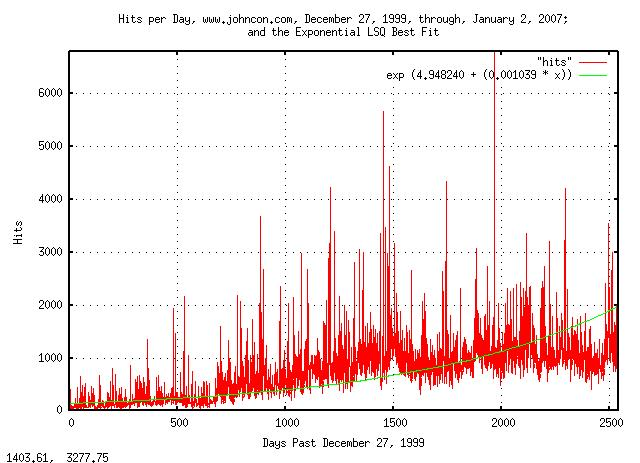Figure XI

Figure XI is a plot of the web server hits per day for domain www.johncon.com, from December 27, 1999, through, January 2, 2007, and its median value, determined by exponential LSQ best fit. (The hits were filtered to exclude crawlers and information robots.)

And, analyzing the increments of the server hits:

``````

tsmath -l "hits" | tslsq -o | tsderivative | tsnormal -t > "hits.distribution"
tsmath -l "hits" | tslsq -o | tsderivative | tsnormal -f -t > "hits.frequency"

``````

And plotting: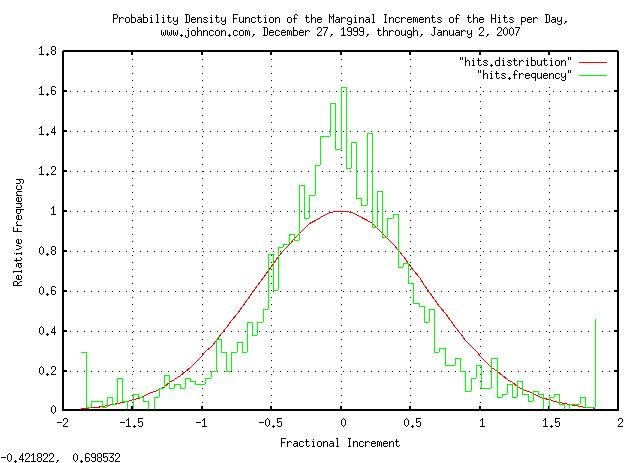Figure XII

Figure XII is a plot of the distribution of the marginal increments of the Brownian Motion, (random walk,) equivalent of the web server hits per day for domain www.johncon.com, from December 27, 1999, through, January 2, 2007, which should be compared with Figure III, above.

Note the implications of the analysis:

• The page hits of the web server sites on the Internet will evolve into a log-normal distribution.

• The duration of time (i.e., the median time,) that a site is the most popular, as measured by the number of hits per day, will be `erf (1 / sqrt (t))`, or a little over 4 years, (using years as the time scale.)

• The ratio of the number of hits per day of the most popular site to the median of all sites will diverge as ```e^sqrt (t)``` over time.

• The growth in the number of page hits per day will grow exponentially, (although the exponential rate will vary, randomly-even decreasing at times.)

### Appendix II, The DJIA, Revisited

Black Scholes Merton methodology can be used to estimate the severity of a downturn in the markets. The methodology assumes the paradigm that equity prices are a random walk fractal, i.e., starting at any specific time, a Gaussian/Normally distributed random number, (with a standard deviation of about 1% = 0.01, of the current price,) is added to the current price of the equity to get the next day's price, and then a second random number is added to get the third day's price, and so on. (Note that the market's value, over time, is a sum of Gaussian/Normally distributed random numbers under this paradigm.)

For details, see: Section I, Section II, Section III, Section IV, Section V,Section VI and Addendum of this series.

Under this paradigm, the equity's price will have a standard deviation, at some time `t` in the future, of `0.01 * sqrt (t)`. What this means for, say, `t = 100` days is that the equity's price will be within one standard deviation, (```0.01 * sqrt (100) = 100%``` = +/- 50%,) 68% of the time. This is the statistical metric of the magnitude of an equity's price bubble, (be it gain, or loss, in value.)

Further, under this paradigm, the chances of the duration of an equity's price being above, (or below,) its value at a specific time for at least `t` many days in the future is `erf (1 / sqrt (t))`, which is about `1 / sqrt (t)` for ```t >> 1```. What this means is that for, say, for at least `t = 100` days in the future, the chances of an equity's price being above, (or below,) its starting price will be ```1 / sqrt (100) = 0.1 = 10%```. This is a statistical metric of the duration of an equity's price bubble, (be it gain, or loss, in value.)

Using the daily closes of the DJIA, (from Yahoo! Finance, ticker ^DJI,) and cutting out the three major declines of the DJIA in the last century, (starting on September 3, 1929; January 14, 2000; October 9, 2007,) and normalizing to the DJIA's value on October 9, 2007, (i.e., all start at 14,164.53,) to compare the declines: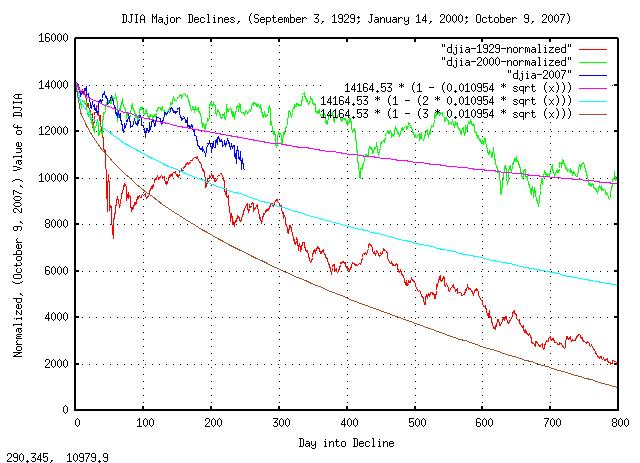Figure XIII

Figure XIII is a plot of the DJIA major declines, September 3, 1929; January 14, 2000; October 9, 2007, and, the one, two, and, three standard deviations in the magnitude of the DJIA's contractions, which was found from:

``````

tsfraction djia | tsavg -p
0.000230
tsfraction djia | tsrms -p
0.010954

``````

Note that the September 3, 1929, decline, (i.e., during the Great Depression,) was about a 2.5 sigma event, (which is about a 1 in 161 chance in any 800 day period.) The January 14, 2000, decline, (i.e., the dot com bubble crash,) was about a 0.625 sigma event, (which has about a 1 in 3.75 chance in any 800 day period.) Extrapolating, it looks like the current financial crisis, (October 8, 2007,) is about a 1.25 sigma event, (which is about a 1 in 9.46 chance in any 800 day period, if it continues.) It would appear, that if the crisis continues, it will be about twice as bad as the January 14, 2000, decline, and about half as bad as the September 3, 1929 decline.

There is a 50% chance, (```0.5 = erf (1 / sqrt (4.4)```,) that the current crisis, (October 8, 2007,) will continue at least 4.4 years from October 8, 2007. The chances of it lasting at least a decade, (```32% = 0.32 = 1 / sqrt (10)```,) and so on.

So, how bad was the Great Depression?

• On September 3, 1929, the DJIA's value was 381.17, the highest until November 23, 1954, when it was 382.74.

• On July 8, 1932, the DJIA's value had deteriorated to 41.22, a loss of 89% in value.

• During the interval of the decline, (September 3, 1929, to, July 8, 1932,) asset, (including housing,) deflation was about 60%.

• During the interval of the decline, the US GDP declined about 40%

• In 1932, about 1 in 4 was unemployed

Note that the equity markets, asset values, US GDP, etc., all tend to track, (but at different rates,) so an assessment can be made, assuming the current crisis continues, and it will be about half as bad as the Great Depression. The chances of the current crisis lasting half as long as the Great Depression, (```(1954 - 1929) / 2 = 12.5```,) is ```1 / sqrt (12.5) = 0.28 = 28%``` which is about 1 chance in 4, (and a 1 chance in 2 of it lasting 4.4 years.)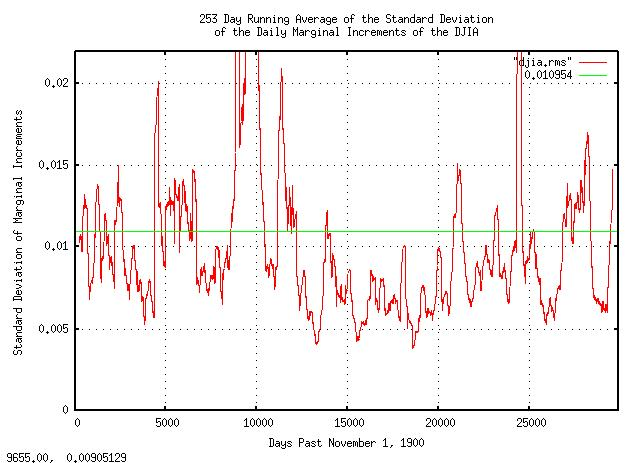Figure XIV

Figure XIV is a plot of the 253 day running average of the standard deviation of the daily marginal increments of the DJIA, from November 1, 1900, to October 3, 2008. Note that it was abnormally large in every decline of the DJIA. Observe how the standard deviation of the daily marginal increments effect the following equations:

``````

avg
--- + 1
rms
P = ------- ........................................(1.24)
2

P          (1 - P)
g = (1 + rms)  (1 - rms)        ....................(1.20)

``````

Where `avg` is the arithmetic average of the marginal increments of an equity market's value, `rms` is the standard deviation of the marginal increments, `P` is the probability of an up movement in the marginal increments, (i.e., the chances a marginal increment will be greater than unity,) and, `g` is the average gain of the marginal increments. (See: Important Formulas for specifics, and, Section I of this series for the derivation of the equations.)

Note that `rms` effects `P`, which is the exponent in `g`, (`avg` varies, too-in the opposite direction of `rms`, but it is not as dramatic.) In point of fact, if `rms` is double its long term value, `g` will be in decline, (i.e., be less than unity.) This is the mechanism of market declines, (and many professional traders use it as a forecasting method for potential declines and bottoms.) For example, the second largest calendar year gain in the DJIA was 1933, (69.2697%-right in the middle of the Great Depression-the largest was 1915, 80.8713%.) Note, also, that the `erf (1 / sqrt (t))` probability of a bubble's duration means that there is a 50/50 chance of the duration being longer than 4.4 years, etc., (for example, on July 17, 1990, with a value of 2999.76, the DJIA deteriorated to 2365.10 on October 11, 1990-in 62 trading days following a three sigma decline-then recovered in the next 160 trading days, on May 30, 1991.) So, `rms` is the mechanism of market gains, too. (The `rms` can be too small-there is an optimal value, in relation to the `avg`, see: Section I.)

In this context, the statement "the current 2008 crisis is a 1.25 sigma event, (i.e., about twice as bad as the 2000 market decline, and, half as bad as the Great Depression, for perspective,) and there is a 50/50 chance that it will be over before 4.4 years from October, 2007, and a 1 in 4 chance that it will last at least 12.5 years," makes reasonable sense.

Note, also, `rms` is a metric of risk, and represents the volatility of the equity market, (its also called a metric of greed by pundits, too.) It is, also, inversely proportional to confidence in the market, and is the engine of market bubbles, (in gain, or loss.)

A note about this section. In an effort to keep things simple, traditional Black Scholes Merton methodology was used, which is adequate for short term projections. However, Section III of this series offers a similar, (it uses the same principles,) methodology that is substantially more accurate, in the long term.

### Appendix III, Regression Studies in Non-Linear High Entropy Economic Systems

The prevailing wisdom is that economic systems are mathematically deterministic and the concepts of classical physics can be used for analysis-such as regression and correlation studies.

A short disproof by contradiction is in order. By building a very precise simulation of the characteristics of a non-linear dynamical high entropy economic system with an average of the marginal increments, `avg = 0.0004`, and standard deviation of the marginal increments of ```rms = 0.02```, meaning the system will have an average increase of 0.04% per day, but it will fluctuate, (with a Gaussian/normal distribution,) of 2% per day, i.e., the fluctuations will be between +/- 2% per day, 68% of the time. These numbers are optimal, (`rms^2 = avg`,) in the sense that the growth is maximum, (increasing, or, decreasing, avg, or increasing, or, decreasing rms, results in lower growth,) and represent the median values of all equities on all exchanges in the US markets in the 20'th Century, (about a hundred thousand of them,) most of the developed countries GDPs, precious metal prices, currency values and exchange rates, commodity prices, and, asset prices, (like housing,) etc. The simulation will be provided by the `tsinvestsim` program from the NtropiX site.

Calculating `P`, the probability of an up movement on any given day:

``````

avg
--- + 1
rms
P = ------- ........................................(1.24)
2

P = 0.51

``````

The control file for the `tsinvestsim` program, `example.0004.02`:

``````

example f = 0.02, p = 0.51

``````

And simulating:

``````

tsinvestsim example.0004.02 90000 | cut -f3 > example.0004.02.ticker

``````

And analyzing:

``````

tsfraction example.0004.02.ticker | tsavg -p
0.000402
tsfraction example.0004.02.ticker | tsrms -p
0.019936

``````

Which are within a percent of what they should be. And, plotting: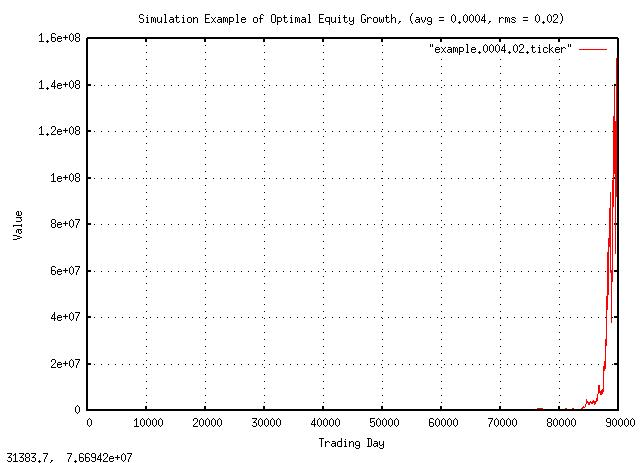Figure XV

Figure XV is a plot of the optimal equity growth simulation. The growth is about a factor of 1.0002 per day, which is about 5% per year, (the simulation is for 90,000 days-about 356 years-to provide a large data set for numerical accuracy.) Note that there are 51 up movements every hundred days, on average, and the average increase per day, 0.0004, is greater than zero; these two number provide the growth in value.

But is it always true?

Changing the metric of risk, ```rms = 0.03``` by 1%, (an increase of 50%,) and calculating `P`, the probability of an up movement on any given day:

``````

avg
--- + 1
rms
P = ------- ........................................(1.24)
2

P = 0.506666666

``````

The control file for the `tsinvestsim` program, `example.0004.03`:

``````

example f = 0.03, p = 0.506666666

``````

And simulating:

``````

tsinvestsim example.0004.03 90000 | cut -f3 > example.0004.03.ticker

``````

And analyzing:

``````

tsfraction example.0004.02.ticker | tsavg -p
0.000404
tsfraction example.0004.02.ticker | tsrms -p
0.029921

``````

Which are, again, within a percent of what they should be. Note there are still more up movements every hundred days, (50.7-about 3 per 510 less than before, but still more,) on average, and the average increase per day, 0.0004, is what it was before, (and is greater than zero.)

And plotting: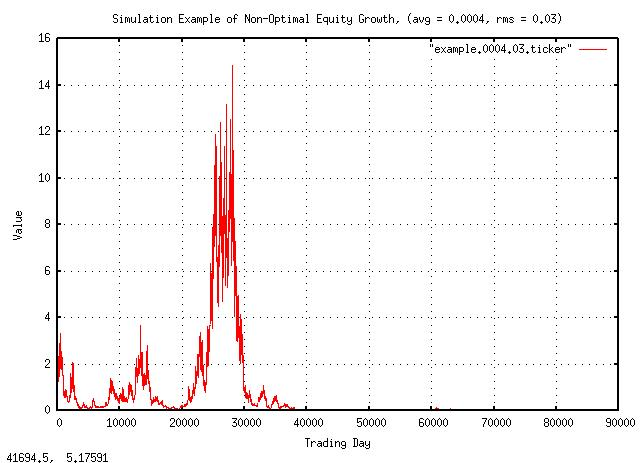Figure XVI

Figure XVI is a plot of the non-optimal equity growth simulation. The growth is negative; after building to a value of 15, the equity goes bust about half way through the simulation. Compare with the previous simulation when at 356 years, the equity was worth about \$140 million!

It is, indeed, counter intuitive that a stock that moves up more times than it goes down, and has a positive average gain, can deteriorate in value to nothing.

Here is what happened, in detail.

The first simulation, (optimal equity value growth):

``````

P          (1 - P)
g = (1 + rms)  (1 - rms)        ....................(1.20)

0.51           (1 - 0.51)
g = (1 + 0.02)     (1 - 0.02)

0.51     0.49
g = 1.02     0.98

g = 1.0101505 * 1.009750517

g = 1.0002

``````

Which is positive growth. And, for the second simulation, (non-optimal equity value growth):

``````

P          (1 - P)
g = (1 + rms)  (1 - rms)        ....................(1.20)

0.506666666           (1 - 0.506666666)
g = (1 + 0.03)            (1 - 0.03)

0.506666666     0.493333334
g = 1.03            0.97

g = 1.01508917 * 0.9850858

g = 0.99995

``````

Which is negative growth.

The intuitive interpretation of the regression analysis was misleading.

Things that fluctuate up more than they fluctuate down, and have a positive average daily gain, do not always increase in value; and the numbers in both simulations are very representative of real world economic phenomena like GDP, equity growth, precious metal values, housing prices, commodity prices, inflation, etc.

### Appendix IV, Comparison of the Laplacian and Normal/Gaussian Probability Distributions of the Marginal Increments in Financial Time Series

The Laplacian Probability Distribution of the marginal increments is ubiquitous in financial time series, (for example, the DJIA, Figure III, above.) The distribution is most pronounced in high speed time series, (day trading, and shorter,) and the deviation of the marginal increments is projected, (via the Central Limit Theorem,) to estimate the Normal/Gaussian probability distribution of the value of an equity, (or other financial instrument,) at some future date-which is quite accurate in the long run.

However, in the short run, the methodology can lead to very optimistic risk assessments.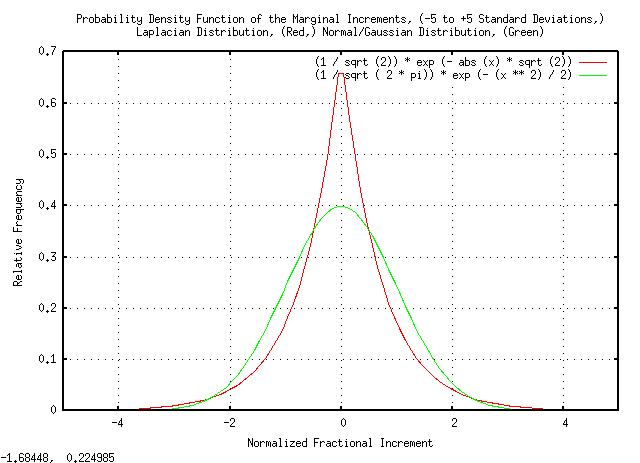Figure XVII

Figure XVII is a plot of the Laplacian and the Normal/Gaussian probability distributions, both normalized to unity standard deviation, from -5 standard deviations to +5 standard deviations.

Note there are substantially more small increments in the Laplacian than the Normal/Gaussian distribution below one standard deviation. And, there are more large increments in the Normal/Gaussian than the Laplacian distribution between one and two standard deviations.

Expanding the plot for better visibility above 2 standard deviations: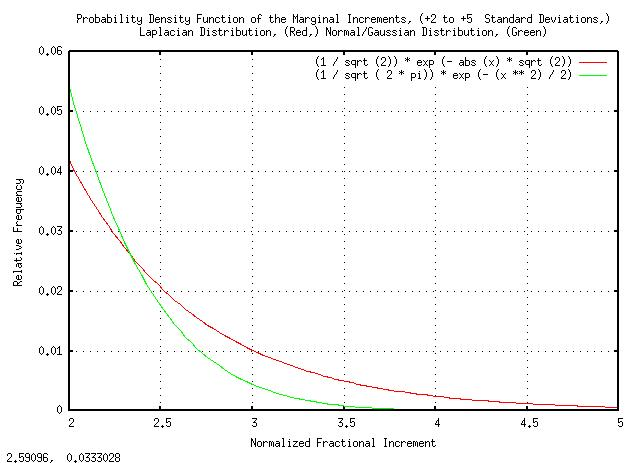Figure XVIII

Figure XVIII is a plot of the Laplacian and the Normal/Gaussian probability distributions, both normalized to unity standard deviation, from +2 standard deviations to +5 standard deviations.

Note that the frequency of 3 standard deviation increments in the Laplacian is about twice that of the Normal/Gaussian probability distribution-a very substantial error in risk estimation when the value of the risk should be known to better than 1% accuracy.

 As a side bar, Black Swans do exist in financial time series. What's the frequency of seven standard deviation movements in the daily increments of financial time series using a Normal/Gaussian probability distribution? About once every three billion years. And, the frequency of seven standard deviation movements for the Laplacian probability distribution? More than once a century. Interestingly, the cumulative distribution function of the Laplacian probability distribution is in very reasonable agreement with the number and magnitude of large increment Black Swan movements in the DJIA, Figure III, analyzed, above. The point is that, although the Normal/Gaussian probability distribution/Central Limit Theorem can be a very accurate methodology for estimating the distribution of asset values in the long term, one has to survive the short term Black Swan incremental movements first-and the risks are actually much higher than predicted by the Normal/Gaussian distributed short term increments assumption.
```
--

John Conover, john@email.johncon.com, http://www.johncon.com/

```